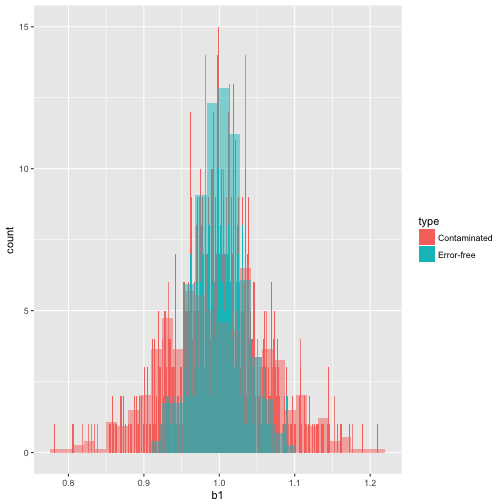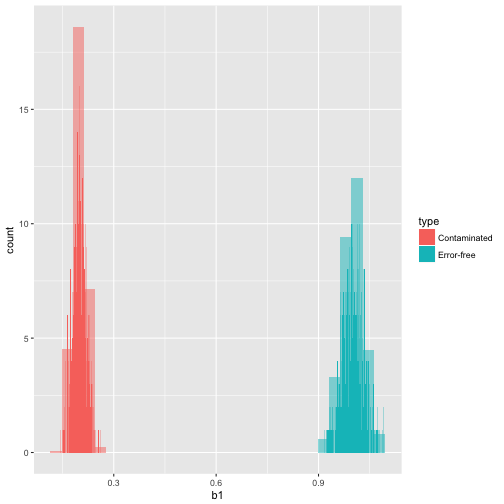This brief tutorial will cover the basics of Monte Carlo Simulations and all the tools that are needed to solve PSet 5. I will use as an example the errors-in-variables model in the context of linear regression. In particular, we want to illustrate with our experiments what we know from studying the theory, as well as some other aspects of programming in R that were left unsaid in the previous tutorial, such as loops, probability distributions, and graphics in R.

# Monte Carlo Experiments

Monte Carlo Experiments are a broad class of computational algorithms that rely on repeated random sampling to obtain numerical results. Their essential idea is using randomness to solve problems that might be deterministic in principle. Monte Carlo methods are mainly used in three distinct problem classes:

1. Generating draws from a probability distribtion
2. Numerical integration
3. Optimization

in this tutorial, we will cover the very basics of the first point, i.e. the possibility of producing a supposedly endless flow of random variables (numbers) for well-known or new distributions.

# Probability Distributions

One convenient use of R is to provide a comprehensive set of statistical tables. Functions are provided to evaluate the cumulative distribution function $$F_X(x)=\mathbf{P}(X\le x)$$, the probability density function of $$F_X(\cdot)$$, $$f_X(\cdot)$$ and the $$t$$-th quantile function, $$F_X^{-1}(\tau)=\inf\{x: F_X(x)>\tau\}$$, and to simulate from the distribution. The following table contains the base functions for a list of distribution functions:

Beta beta shape1, shape2, ncp
Binomial binom size, prob
Cauchy cauchy location, scale
Chi-Squared chisq df, ncp
Exponential exp rate
F f df1, df2, ncp
Gamma gamma shape, scale
Geometric geom prob
Hypergeometric hyper m, n, k
Log-normal lnorm meanlog, sdlog
Logistic logis location, scale
Negative Binomial nbinom size, prob
Normal norm mean, sd
Poisson pois lambda
Signed Rank signrank n
t t df, ncp
Uniform unif min, max
Weibull weibull shape, scale
Wilcoxon wilcox m, n

The typical use is the following: if you're interested in the density of a given distribution, add the letter d at the beginning of the base function. In this case, the function calls for argument x. For instance for the standard normal,

    dnorm(x=1.64)

 0.1039611


If you are interested in the CDF, add the letter p at the beginning. The argument is a real number, x. Again, if the probability distribution of choice is the standard normal,

    pnorm(q=0)

 0.5


Similarly, q for the quantile function. In this case the argument is a probability, p,

    qnorm(p=0.05)

 -1.644854


and finally, r for a draw from the specified distribution. The argument is the number of draws (sample size), n:

    rnorm(n=5)

 -0.27491552 -0.76909794 -0.80557494  0.06713161 -1.85387339


These rules apply to all the base function in the table above, however if you want to draw a random number from rhyper, rsignrank or rwilcox, the correct argument is nn, rather than n.

# Loops and Conditional Statements

## if Statement

The conditional construction follows the form if(exp 1){ exp 2 }else{ exp3}. For instance

    # Generate a random number in [0,1]
r.number <- runif(1)
# Loop
if(r.number>0.3){
print("ok number")
}else {
print("small number")
}

 "small number"


There is a vectorized version of the if/else construct, the ifelse function. Using previous example,

    # Loop
ifelse(r.number>0.3,"ok number","small number")

 "small number"


## Repetitive execution: for and while

There is also a for loop construction. For a loop variable say i in a vector expression (most frequently, a sequence), an expression is repeatedly evaluated. For instance,

    for(i in seq(1:5)){
i^2
}


Other looping commands are repeat and while.

# The Model

Let's summarize this model by saying the unobservable variable of interest is $$Z^{*}$$, and its observed but mismeasured counterpart is $Z=Z^{*}+\eta$ where $$\nu$$ denotes the measurement error. Classical Measurement Error assumes that 1) $$Z^{*}$$ and $$\eta$$ are independent, and 2) $$\mathbb{E}(\eta)=0$$.

The textbook example (and conclusion) shows that error in the dependent variable is generally ignored in regression analysis because it simply gets absorbed into the error residual. The only sensible effect is an increase in variability. Hence, inference is more conservative but remains valid nonetheless.

For instance, consider underlying DGP (multivariate normal distribution)

$W= \begin{pmatrix} Y \ X \ \eta \end{pmatrix}=\mathcal{N}_3\left(\mathbf{0}, \begin{pmatrix} \sigma^2_Y & \sigma_{Y,X} & 0 \ \sigma_{X,Y} & \sigma^2_X & 0 \ 0 & 0 & \sigma^2_{\eta} \end{pmatrix}\right)$

and you observe

\begin{align*} Y^{*}&=Y+\eta\ X^{*}&=X+\eta\ \end{align*} Suppose we're interested in parameter $\beta=\frac{\sigma_{Y,X}}{\sigma_{X}^2}=\frac{\mathbf{E}(YX)}{\mathbf{E}(X^2)}$

Draw a random sample of size $$n$$. We consider estimators \begin{align*} \hat{\beta}_1&=\frac{\sum_{i=1}^nX_iY^{*}_i}{\sum_{i=1}^nX_i^2}\:\:\: \text{ and }\:\:\: \hat{\beta}_2=\frac{\sum_{i=1}^nY_iX^{*}_i}{\sum_{i=1}^n (X^{*}_i)^2} \end{align*} i.e. in model 1, $$Y$$ is mismeasured whereas in model 2 variable $$X$$ is mismeasured. It's very easy to show that $$\hat{\beta}_1$$ is unbiased and consistent estimator (recall Pset 0). However, $\hat{\beta}_2\overset{P}{\rightarrow}\beta\left(\frac{\sigma^2_X}{\sigma^2_X+\sigma^2_{\eta}}\right)\le\beta \:\:\:\text{ as }\:\:\:n\to\infty$

We can illustrate this with a simple Monte Carlo experiment:

1. Draw a sample of size $$1000$$ from the underlying multivariate normal distribution with parameters $$\sigma^2_y=1$$, $$\sigma_x^2=0.5$$, $$\sigma_{\eta}^2=2$$, and $$\sigma_{y,x}=0.5$$.
2. Generate $$Y^{*}$$ and $$X^{*}$$ as described earlier.
3. Calculate $$\hat{\beta}_1$$ and $$\hat{\beta}_2$$.
4. Repeat previous steps $$500$$ times.

Then the parameters of the DGP

mu <- c(0,0,0)
sigma_y <- 1
sigma_x <- 0.5
sigma_e <- 2
rho=0.5
sigma_yxe <- matrix(c(sigma_y,rho,0,rho,sigma_x,0,0,0,sigma_e),ncol=3)


Parameter of interest

    b <- rho*sqrt(sigma_y/sigma_x)


Sample size

    N <- 1000


Number of simulations

    n.sims <- 500


Last but not least, the seed. The seed will allow us to replicate and reproduce results. This is one of the most important practices in Monte Carlo Experiments

    set.seed(1984)


We want to store 6 estimates: $$\beta_0$$ and $$\beta_1$$ for three models:

1. Without measurement error
2. Only $$Y$$ is measured with error.
3. Only $$X$$ is measured with error.

The store matrix

    out <- matrix(NA,nrow=n.sims,ncol=6)


at each of the 500 replications, we are generating vector $$Z$$

    # Call the package
require(mvtnorm)
Z <- rmvnorm(n=N,mean = mu, sigma = sigma_yxe)


Contaminated data

    Ye <- Z[,1]+Z[,3]
Xe <- Z[,2]+Z[,3]


Estimate the BLP for the three cases

    fit1 <- lm(Z[,1]~Z[,2])
fit2 <- lm(Ye~Z[,2])
fit3 <- lm(Z[,1]~Xe)


Then the 6 coefficients are given by

    one.sim <- c(fit1$coefficients,fit2$coefficients,fit3$coefficients) one.sim   (Intercept) Z[, 2] (Intercept) Z[, 2] (Intercept) 0.009104504 0.988055248 -0.032965339 0.989833654 0.014665308 Xe 0.215282638  If we put this in a loop  for(i in 1:n.sims){ # Data Generating Process Z <- rmvnorm(n=N,mean = mu, sigma = sigma_yxe) # Contaminated Data Ye <- Z[,1]+Z[,3] Xe <- Z[,2]+Z[,3] # Estimating true relationships fit1 <- lm(Z[,1]~Z[,2]) # Let's estimate the slope coefficient # of a linear model ye=bxe+epsilon fit2 <- lm(Ye~Z[,2]) fit3 <- lm(Z[,1]~Xe) out[i,] <- c(fit1$coefficients,fit2$coefficients,fit3$coefficients)
}


Let's create a data frame with the results

    coeff1 <- data.frame(b1  = out[,2])
coeff1$type <- 'Error-free' coeff2 <- data.frame(b1 = out[,4]) coeff2$type <- 'Contaminated'


and combine into your new data frame vegLengths

    betaEst <- rbind(coeff1, coeff2)


Call package ggplot2

    require(ggplot2)


Plot the histograms. First, when only $$Y$$ is mismeasured

    ggplot(betaEst, aes(b1, fill = type)) +
stat_bin(binwidth = 0.001) +
geom_histogram(alpha = 0.5, aes(y = ..density..), position = 'identity')

stat_bin() using bins = 30. Pick better value with binwidth.and then when only $$X$$ is measured with error

    coeff3 <- data.frame(b1  = out[,6])
coeff3\$type <- 'Contaminated'
betaEst <- rbind(coeff1, coeff3)
ggplot(betaEst, aes(b1, fill = type)) +
stat_bin(binwidth = 0.001) +
geom_histogram(alpha = 0.5, aes(y = ..density..), position = 'identity')

stat_bin() using bins = 30. Pick better value with binwidth.